### Example 13.1 Multicommodity Flow Problem

This example demonstrates how to use the decomposition algorithm to find a minimum-cost multicommodity flow (MMCF) in a directed network. This type of problem was motivation for the development of the original Dantzig-Wolfe decomposition method (Dantzig and Wolfe, 1960).

Let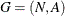be a directed graph, and let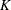be a set of commodities. For each link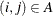and each commodity, associate a cost per unit of flow, designated by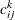. The demand (or supply) at each node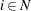for commodityis designated as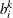, where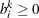denotes a supply node and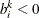denotes a demand node. Define decision variables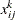that denote the amount of commoditysent from nodeand node. The amount of total flow, across all commodities, that can be sent across each link is bounded above by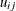.

The problem can be modeled as a linear programming problem as follows: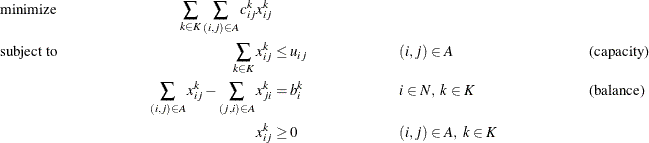In this formulation, constraints (capacity) limit the total flow across all commodities on each arc. The constraints (balance) ensure that the flow of commodities leaving each supply node and entering each demand node are balanced.

Consider the directed graph in Output 13.1.1 which appears in Ahuja, Magnanti, and Orlin (1993).

Output 13.1.1: Example Network with Two Commodities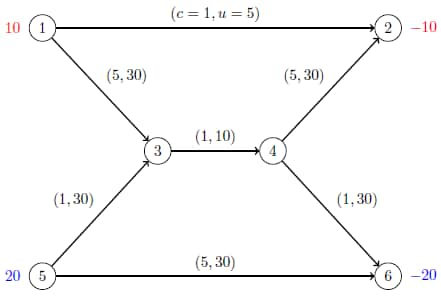The goal in this example is to minimize the total cost of sending two commodities across the network while satisfying all supplies and demands and respecting arc capacities. If there were no arc capacities linking the two commodities, you could solve a separate minimum-cost network flow problem for each commodity one at a time.

The following data set arc_comm_data provides the costof sending a unit of commodityalong arc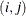:

data arc_comm_data;
input k i j cost;
datalines;
1 1 2 1
1 1 3 5
1 5 3 1
1 5 6 5
1 3 4 1
1 4 2 5
1 4 6 1
2 1 2 1
2 1 3 5
2 5 3 1
2 5 6 5
2 3 4 1
2 4 2 5
2 4 6 1
;


Next, the data set arc_data provides the capacityfor each arc:

data arc_data;
input i j capacity;
datalines;
1 2  5
1 3 30
5 3 30
5 6 30
3 4 10
4 2 30
4 6 30
;


Lastly, the data set supply_data provides the nonzero supply (or demand)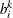for each node and each commodity:

data supply_data;
input k i supply;
datalines;
1 1  10
1 2 -10
2 5  20
2 6 -20
;


The following PROC OPTMODEL statements find the minimum-cost multicommodity flow:

proc optmodel;
set <num,num,num> ARC_COMM;
num cost {ARC_COMM};
read data arc_comm_data into ARC_COMM=[i j k] cost;

set ARCS = setof {<i,j,k> in ARC_COMM} <i,j>;
set COMMODITIES = setof {<i,j,k> in ARC_COMM} k;
set NODES = union {<i,j> in ARCS} {i,j};

num capacity {ARCS};
read data arc_data into [i j] capacity;

num supply {NODES, COMMODITIES} init 0;
read data supply_data into [i k] supply;

var Flow {<i,j,k> in ARC_COMM} >= 0;
min TotalCost =
sum {<i,j,k> in ARC_COMM} cost[i,j,k] * Flow[i,j,k];
con BalanceCon {i in NODES, k in COMMODITIES}:
sum {<(i),j,(k)> in ARC_COMM} Flow[i,j,k]
- sum {<j,(i),(k)> in ARC_COMM} Flow[j,i,k] = supply[i,k];
con CapacityCon {<i,j> in ARCS}:
sum {<(i),(j),k> in ARC_COMM} Flow[i,j,k] <= capacity[i,j];


Because each (balance) constraint involves variables for only one commodity, a decomposition by commodity is a natural choice. In both the OPTLP and OPTMILP procedures, the block identifiers must be consecutive integers starting from. In PROC OPTMODEL, the block identifiers only need to be numeric. The following FOR loop populates the .block constraint suffix with block identifier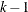for commodity:

   for{i in NODES, k in COMMODITIES}
BalanceCon[i,k].block = k - 1;


The .block constraint suffix for the linking (capacity) constraints is left missing, so these constraints become part of the master problem.

The following SOLVE statement uses the DECOMP= option to invoke the decomposition algorithm:

   solve with LP / presolver=none decomp=() subprob=(algorithm=nspure);
print Flow;
quit;


Here, the PRESOLVER=NONE option is used, because otherwise the presolver solves this small instance without invoking any solver. Because each subproblem is a pure network flow problem, you can use the ALGORITHM=NSPURE option in the SUBPROB= option to request that a network simplex algorithm for pure networks be used instead of the default algorithm, which for linear programming subproblems is primal simplex.

It turns out for this example that if you specify METHOD=NETWORK (instead of the default METHOD=USER) in the DECOMP= option, the network extractor finds the same blocks, one per commodity. To invoke the METHOD=NETWORK option, simply change the SOLVE statement as follows:

  solve with LP / presolver=none decomp=(method=network);


In this case, the default subproblem solver is NSPURE.

The optimal solution and solution summary are displayed in Output 13.1.2.

Output 13.1.2: Solution Summary and Optimal Solution

The OPTMODEL Procedure

Solution Summary
Solver LP
Algorithm Decomposition
Objective Function TotalCost
Solution Status Optimal
Objective Value 150

Primal Infeasibility 0
Dual Infeasibility 0
Bound Infeasibility 0

Iterations 4
Presolve Time 0.00
Solution Time 0.11

   Flow
1 2 1 5
1 2 2 0
1 3 1 5
1 3 2 0
3 4 1 5
3 4 2 5
4 2 1 5
4 2 2 0
4 6 1 0
4 6 2 5
5 3 1 0
5 3 2 5
5 6 1 0
5 6 2 15

The optimal solution is shown on the network in Output 13.1.3.

Output 13.1.3: Optimal Flow on Network with Two Commodities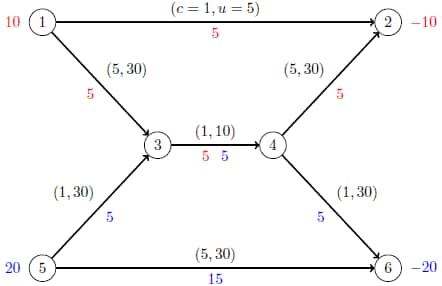The iteration log, which contains the problem statistics, the progress of the solution, and the optimal objective value, is shown in Output 13.1.4.

Output 13.1.4: Log

 NOTE: There were 14 observations read from the data set WORK.ARC_COMM_DATA. NOTE: There were 7 observations read from the data set WORK.ARC_DATA. NOTE: There were 4 observations read from the data set WORK.SUPPLY_DATA. NOTE: Problem generation will use 4 threads. NOTE: The problem has 14 variables (0 free, 0 fixed). NOTE: The problem has 19 linear constraints (7 LE, 12 EQ, 0 GE, 0 range). NOTE: The problem has 42 linear constraint coefficients. NOTE: The problem has 0 nonlinear constraints (0 LE, 0 EQ, 0 GE, 0 range). NOTE: The LP presolver value NONE is applied. NOTE: The LP solver is called. NOTE: The Decomposition algorithm is used. NOTE: The Decomposition algorithm is executing in single-machine mode. NOTE: The DECOMP method value USER is applied. NOTE: The decomposition subproblems consist of 2 disjoint blocks. NOTE: The decomposition subproblems cover 14 (100.00%) variables and 12 (63.16%) constraints. NOTE: The deterministic parallel mode is enabled. NOTE: The Decomposition algorithm is using up to 4 threads. Iter          Best        Master       Gap       CPU      Real Bound     Objective                Time      Time NOTE: Starting phase 1. 1        0.0000       15.0000  1.50e+01       0.0       0.0 3        0.0000        0.0000     0.00%       0.0       0.0 NOTE: Starting phase 2. 4      150.0000      150.0000     0.00%       0.0       0.0 NOTE: The Decomposition algorithm used 2 threads. NOTE: The Decomposition algorithm time is 0.01 seconds. NOTE: Optimal. NOTE: Objective = 150.